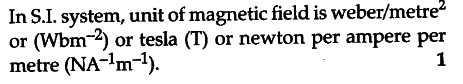# Use the expression F = q(vx B) to define the S.I. unit of magnetic field

The expression for magnetic field is,
F = q(vxB)
If v and B both are normal to each other, then F = qvB.
Now if q = 1, v = 1, then F = B
Hence, the magnetic field at a point is equal to the force which a unit charge experiences when it enters with unit velocity in a direction normal to the direction of the magnetic field at that point.